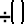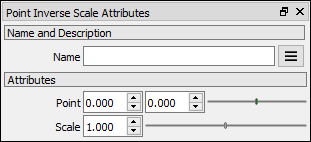# Point Inverse Scale node

Left Toolbar >Math Nodes >Point Inverse Scale

The Point Inverse Scale node allows you to execute an inverse uniform scaling transformation of a point value.

## Procedure

### To execute a point inverse scale operation in the node graph:

1. On the left toolbar choose Point Nodes> Point Inverse Scale2. Drag the Point Inv Scale icon into the Node Graph.3. Connect the point Node to the input.

4. Set the Inverse scale factor.

5. Connect the Output: result .

## Inputs

point A POINT type of value.

scale A NUMBER type of value.

## Outputs

result

A POINT type of value.(x,y)

## Attributes PanelPoint

This attribute is exposed as input by default. Right-click the Node to hide this attribute as input and set the point values manually.

Scale This attribute is exposed as input by default. Value 1. Right-click the Node to hide this attribute as input and set the value manually.

Math Nodes

Main Page